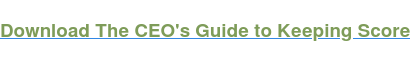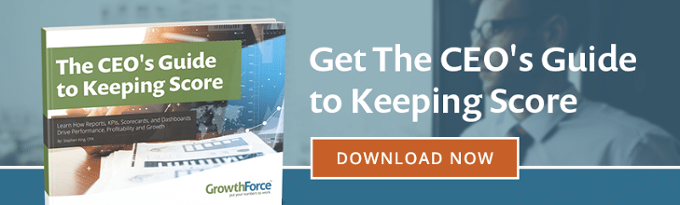# Variance Analysis

Variance analysis measures the differences between expected results and actual results of a production process or other business activity. Measuring and examining variances can help management contain and control costs and improve operational efficiency.

Prior to an accounting period, a budget is made using estimates of material and labor costs and amounts that will be required for the period. After the accounting period, compare the actual material and labor costs and amounts to the estimates to see how accurate the estimates were. The differences between the estimates and the actual results observed at the end of the period are called the variances.

Commonly measured variances include direct labor rate variance, direct labor efficiency variance, direct material price variance, and direct material quantity variance. These variance analyses compare expected results to actual results. The purpose is to see if budget targets were met. Or they see if the operations ended up being more expensive or less costly than originally planned.## Variance Interpretation

Variance analysis will let managers and cost analysts see if the budgeted costs and requirements for an operation accurately forecasted the actual costs and requirements of the operation.

Often, you will find variance between the budgeted requirements and the actual requirements. It is then up to managers and cost analysts to determine if that variance was favorable or unfavorable.

When a variance is favorable, that means that the actual costs and requirements of the operations were less than the expected costs and requirements for the operations. In other words, they expected the production process to cost a certain amount and it ended up costing less. Hence, this is a favorable variance.

When a variance is unfavorable, that means that the actual costs and requirements of the operations were more than the expected costs and requirements for the operations. In other words, they expected the production process to cost a certain amount and it ended up costing more. In conclusion, this is an unfavorable variance.### Source:

Hilton, Ronald W., Michael W. Maher, Frank H. Selto. “Cost Management Strategies for Business Decision”, Mcgraw-Hill Irwin, New York, NY, 2008.